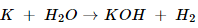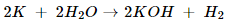### Chemical Reactions and Equations - Solutions

CBSE Class 10 Science

NCERT Solutions
Chapter - 1
Chemical reactions and equations

(Page No. 6)

1. Why should a magnesium ribbon be cleaned before burning in air?

Ans. Magnesium ribbon is cleaned before burning to remove the protective layer of basic magnesium carbonate from the surface of magnesium ribbon.

2. Write the balance equation for the following reactions Give reasons for the following reactions?

i. Hydrogen + Chlorine  Hydrogen chloride

ii. Barium chloride + Aluminium sulphate  Barium sulphate + Aluminium chloride

iii. Sodium + water  Sodium hydroxide + water

Ans. The chemical equations are as follows-

i. H2 + Cl2 $\to$ 2HCl.

ii. 3BaCl+ Al2(SO4)3 $\to$ 3BaSO4 + 2AlCl3.

iii. 2Na + 2H2$\to$ 2NaOH + H2.

3. Write the balanced chemical equation with state symbols for the following reactions?

i. Solutions of barium chloride and sodium sulphate in water react to give insoluble barium sulphate and solution of sodium chloride.

ii. Sodium hydroxide solution (in water) reacts with hydrochloric acid solution (in water) to produce sodium chloride solution and water.

Ans. Balance chemical reaction with state symbols are as follows-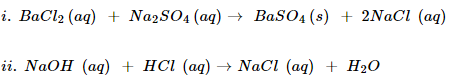(Page No. 10)

1. A solution of a substance ‘X’ is used for white washing

i. Name the substance ‘X’ and writes its formula.

ii. Write the reaction of the substance ‘X’ named in (i) above with water

Ans. i. The substance whose solution is water is used for white washing is calcium oxide. Its formula is CaO.

ii. CaO(s) + H2O(l) $\to$ Ca(OH)2(aq)

2. Why the amount of gas collected in one of the tubes in Activity 1.7 double of the amount collected in the other? Name this gas.

Ans. The gas which is collected in double the amount in the electrolysis of water experiment is hydrogen. This is because water contains 2 parts of hydrogen element as compared to only 1 part of oxygen element.

(Page No. 13)

1. Why does the colour of copper sulphate solution change when an iron nail is dipped in it?

Ans. When iron nail is dipped in copper sulphate solution, than iron sulphate solution and copper solution and copper metal are formed: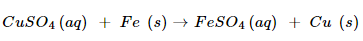In this reaction, iron displaces copper from copper sulphate solution. The deep blue colour of copper sulphate fades due to the formation of light green solution of iron sulphate.

2. Give an example of a double displacement reaction other than the one given in Activity 1.10.

Ans. An example of double displacement reaction is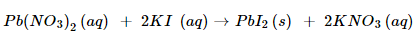3. Identify the substances that are oxidized and the substances that are reduced in the following reactions.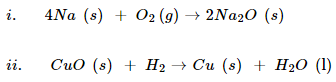Ans. (i).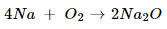In this reaction, Na is oxidized because it combines with O2 to form Na2O. O2 is reduced because it is converted into Na2O.

(ii).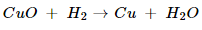In this reaction, CuO is reduced because it loses oxygen. H2 is oxidized because it combines with oxygen of CuO to form water

Textbook exercises

1. Which of the following statement about the reaction below are incorrect?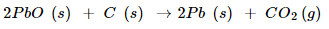(b) Carbon dioxide is getting oxidized

(c) Lead oxide is getting oxidized

i. (a) and (b)

ii. (a) and (c)

iii. (a), (b) and (c)

iv. All

Ans. As statement (a) and (b) are incorrect, answer (i) is correct.

2. $F{e}_{2}{O}_{3}+2Al\to A{l}_{2}{O}_{3}+2Fe$

The above reaction is an example of a

(a) combination reaction

(b) double displacement reaction

(c) decomposition reaction

(d) displacement reaction

Ans. This is an example of displacement reaction because Fe in FeO3 has been displaced by Al. Hence correct answer is (d).

3. What happens when dilute hydrochloric acid is added to iron filling? Tick the correct answer

(a) Hydrogen gas and iron chloride are produced.

(b) Chlorine gas and iron hydroxide are produced

(c) No reaction takes place

(d) Iron salt and water are produced

4. What is balanced chemical equation? Why should chemical equation be balanced?

Ans. The reaction in which the number of atoms of each element is equal on the reactant side and product side is called balanced equation.

Chemical reaction should be balanced because only a balanced equation tells us the relative quantities of different reactants and products involved in the reaction.

5. Translate the following statements into chemical equations and then balance them.

(a) Hydrogen gas combines with nitrogen to form ammonia.

(b) Hydrogen sulphide gas burns in air to give water and Sulpher dioxide.

(c) Barium chloride reacts with aluminum sulphate to give aluminum chloride and precipitate of barium sulphate

Ans. (a).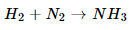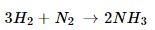(b).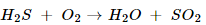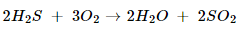(c).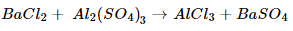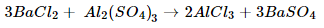(d).